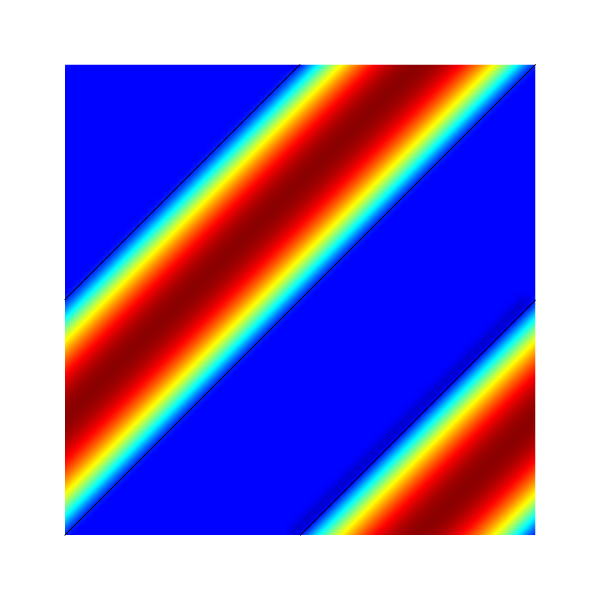# Poiseuille flow in a periodic channel inclined at 45 degrees

We test the embedded boundaries by solving the viscous flow driven by gravity in an inclined periodic channel.

#include "embed.h"
#include "navier-stokes/centered.h"
#include "view.h"

int main()
{
origin (-0.5, -0.5);
periodic (right);
periodic (top);

stokes = true;
TOLERANCE = 1e-7;

for (N = 16; N <= 64; N *= 2)
run();
}

scalar un[];

#define WIDTH 0.5
#define EPS 1e-14

event init (t = 0) {

The gravity vector is aligned with the channel and viscosity is unity.

  const face vector g[] = {1.,1.};
a = g;
mu = fm;

The channel geometry is defined using the levelset function \phi.

  vertex scalar phi[];
foreach_vertex()
phi[] = difference (union (y - x - EPS, x - y - 0.5 + EPS),
y - x - 0.5 + EPS);
boundary ({phi});
fractions (phi, cs, fs);

The boundary condition is zero velocity on the embedded boundaries.

  u.n[embed] = dirichlet(0);
u.t[embed] = dirichlet(0);

We use “third-order” face flux interpolation.

  for (scalar s in {u})
s.third = true;

We initialize the reference velocity.

  foreach()
un[] = u.y[];
}

We check for a stationary solution.

event logfile (t += 0.1; i <= 1000) {
double du = change (u.y, un);
if (i > 0 && du < 1e-6)
return 1; /* stop */
}

We compute the error and display the solution using bview.

event profile (t = end) {
printf ("\n");
foreach()
fprintf (stdout, "%g %g %g %g %g\n", x, y, u.x[], u.y[], p[]);
scalar e[];
foreach() {
double x1 = y - x;
e[] = u.x[] - 0.25*(sq(WIDTH/2.) - sq(x1 >= 0. ? x1 - 0.25 : x1 + 0.75));
}
norm n = normf (e);
fprintf (stderr, "%d %.3g %.3g %.3g %d %d %d %d %d\n",
N, n.avg, n.rms, n.max, i, mgp.i, mgp.nrelax, mgu.i, mgu.nrelax);

draw_vof ("cs", "fs");
squares ("u.x", linear = true, spread = -1);
save ("u.x.png");
}Velocity field

The method is almost exact.

set xlabel 'Resolution'
set ylabel 'Maximum error'
set logscale
set xtics 4,2,128
set ytics format "% .0e"
plot 'log' u 1:4 w lp t ''Error as function of resolution (script)